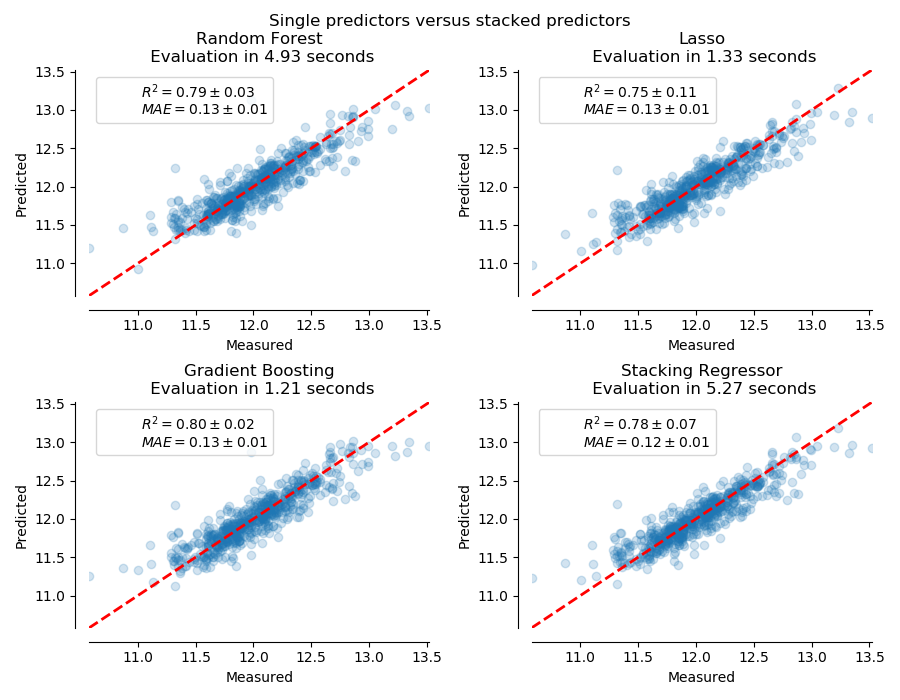# sklearn.model_selection.cross_val_predict¶

sklearn.model_selection.cross_val_predict(estimator, X, y=None, *, groups=None, cv=None, n_jobs=None, verbose=0, fit_params=None, pre_dispatch='2*n_jobs', method='predict')

[源码]

estimator estimator object implementing ‘fit’ and ‘predict’

X array-like of shape (n_samples, n_features)

y array-like of shape (n_samples,) or (n_samples, n_outputs), default=None

groups array-like of shape (n_samples,), default=None

cv int, cross-validation generator or an iterable, default=None

- None，默认使用5折交叉验证
- int，用于指定(Stratified)KFold的折数
- CV splitter,
- 可迭代输出训练集和测试集的切分作为索引数组

n_jobs int, default=None

verbose int, default=0

fit_params dict, defualt=None

pre_dispatch int or str, default=’2*n_jobs’

- None,在这种情况下，所有CPU内核都将立即创建并产生，使它进行轻量级和快速运行的任务，以避免因按需生成作业而造成延迟
-int,给出所产生的总CPU内核数的确切数量
-str,根据n_jobs给出表达式，如'2*n_jobs'
method str, default=’predict’

predictions ndarray

cross_val_score 计算每个交叉验证切分的准确率

cross_validate 计算每个交叉验证切分的一个或多个准确率和时间

>>> from sklearn import datasets, linear_model>>> from sklearn.model_selection import cross_val_predict>>> diabetes = datasets.load_diabetes()>>> X = diabetes.data[:150]>>> y = diabetes.target[:150]>>> lasso = linear_model.Lasso()>>> y_pred = cross_val_predict(lasso, X, y, cv=3)

## sklearn.model_selection.cross_val_predict使用示例¶